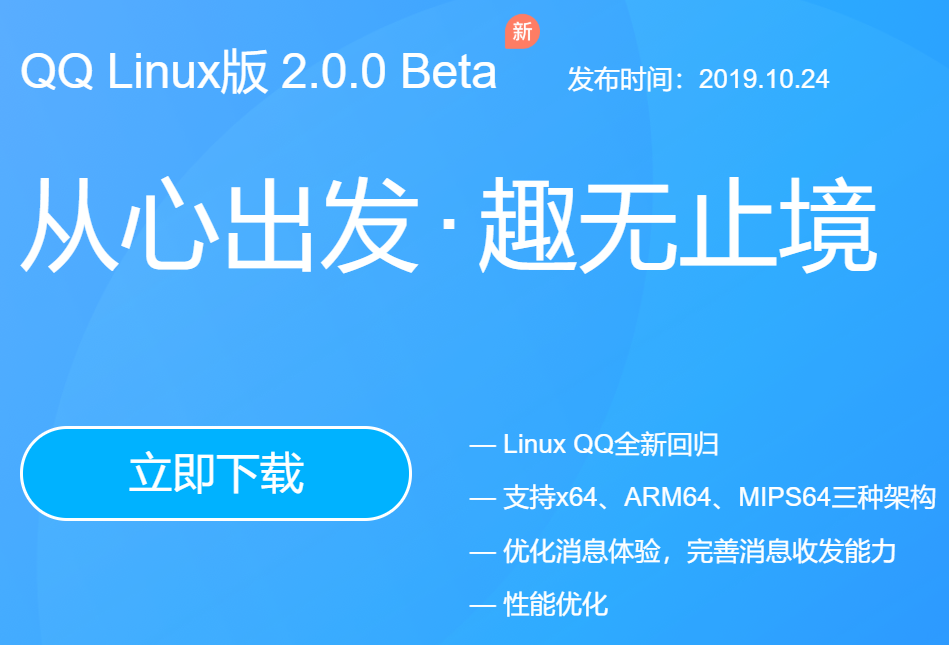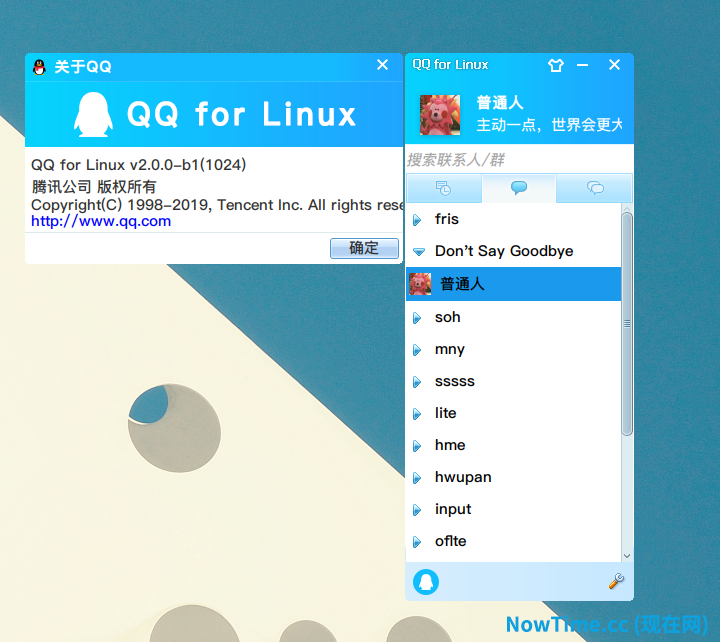# 标号 label

``````char a;
outer:  //this is the label for the outer loop
for(int i=0;i<10;i++)
{
for(int j=0;j<10;j++)
{
if(a=='b')
break outer;
if(a=='c')
continue outer;
}
}``````

``````System.out.println("100~200 的质数");

int n = 0;

outer:
for (int i=101; i<200; i+=2){
for (int j = 2; j<i; j++){
if(i%j == 0) continue outer;//结束本次外层循环
}

System.out.print(" "+i);;//显示质数
n++;//计算质数个数

if(n<10) continue;//未满10个，则不换行
System.out.println();
n = 0;//重置质数个数
}``````

## [简单体验]QQ for Linux 于 2019-10-24 更新# 简单体验了下

## 先总结

• “基本资料”和“详细资料”只能够查看，不能够修改
• 可以发送(群)文件
• 消息编辑框可以粘贴图片发送
• 消息接收及时
• CPU 占用率、内存使用都比较低，运行流畅
• 支持接收QQ经典表情和新增的一些表情
• 消息窗口切换

1.登陆界面2.关于QQ界面- 阅读剩余部分 -

# 代码

``````/**
* 产生指定长度　０～11的数字，且不重复数字的数组，使用了递归方法
* @author chuwen   <wenzhouchan@gmail.com>
* @param length    指定生成数组的长度，默认为５
* @param arr       默认空白数组，不需要填写
* @returns {Array} 输出数组
*/
function random_num(length=5, arr=[]) {
let random = Math.floor(Math.random()*11)+1, repeat = false;
random = random > 9 ? random.toString() : '0'+random;//将数字转化成字符串。如果小于９，给数字前面补一个零

if(arr.length > length-1) return arr;//达到了指定数组长度，直接输出数组

for(let item in arr){
if(arr[item] === random) return random_num(length, arr);//如果数组中存在与本次随机产生的随机数有相同的，就递归调用
}

arr.push(random);//将产生的随机数，追加进　arr
return random_num(length, arr);//然后进行递归调用
}``````

＃ 运行结果

``````console.log(random_num(５));//运行结果如下
["02", "04", "06", "10", "01"]

console.log(random_num(４));//运行结果如下
["03", "10", "08", "06"]``````